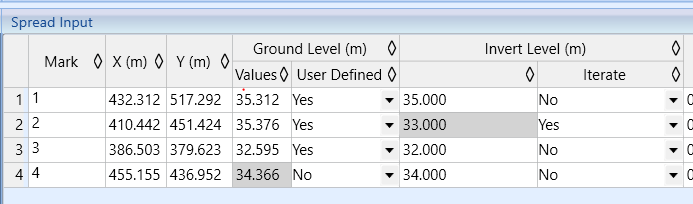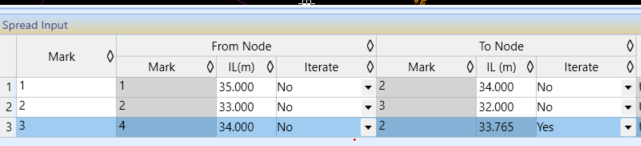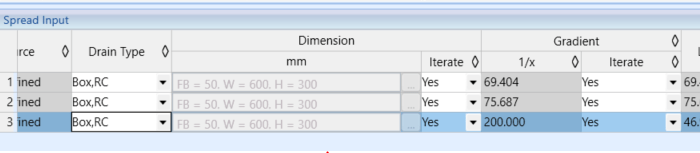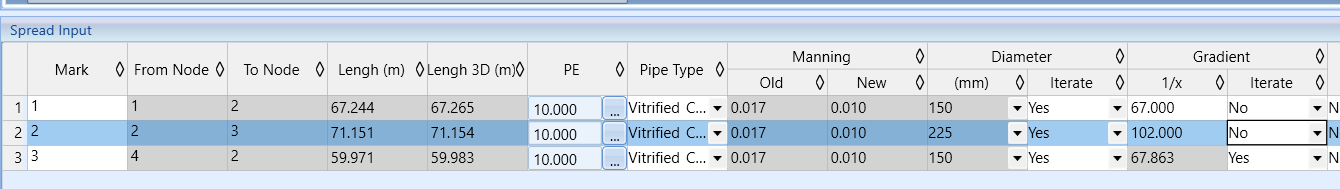603 - 5885 1250 (4 lines) info@mes100.com
Select Page

# Drainage and Sewerage Benchmark per Pipe Basis

Users might have encountered a situation whereby at one node, one needs to manually set the different invert levels at different pipe ends . Such a situation typically arises when engineers need to readjust back the pipe invert level/gradient to tally with on-site condition.

MiTS currently provides users to insert the invert level per pipe basis (upstream and downstream of the pipe, instead of at one node), which then calculate the gradient and drop depth for you. The following examples illustrate and compare the new and old versions.

# DRAINAGE #

## Previous version #Above is how the previous versions work, whereby users have to first calculate the invert level manually, in case where drops is required. Since the invert level is user-defined, thus the gradient also has to be manually calculated in order to adjust the following sump invert level.Gradient is manual calculated and insert the value in the spread input.Image above is the drainage taken from the previous version project. As you notice, users now can straight away insert the invert level of the upstream and downstream of the pipe, without the hurdle of calculating the drop and gradient manually.You can set the gradient iteration to “Yes” as software will calculate based on the invert level assigned at the upstream and downstream of a pipe.

## Benchmark#

We also provide a benchmark to compare between manual calculation with the calculation of the software.

# SEWERAGE#

Sewerage module also works the same as drainage, where users can determine the invert level based on pipe basis. Users no longer need to calculate the drop, gradient and invert level manually.

## Previous version #Previous version requires users to insert the invert level and calculate the gradient manually if certain invert level of sump at a pipe is required.Above is an example of the previous version where the value of gradient needs to be first manually calculated before insert it in the spread input.